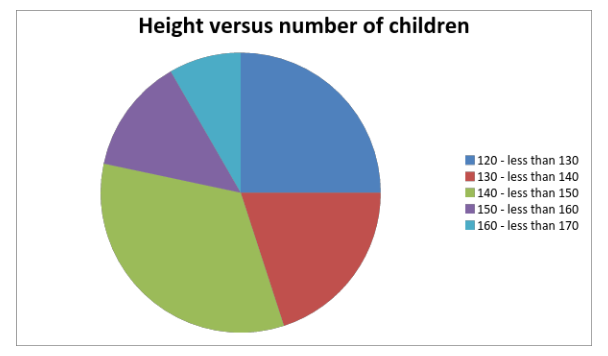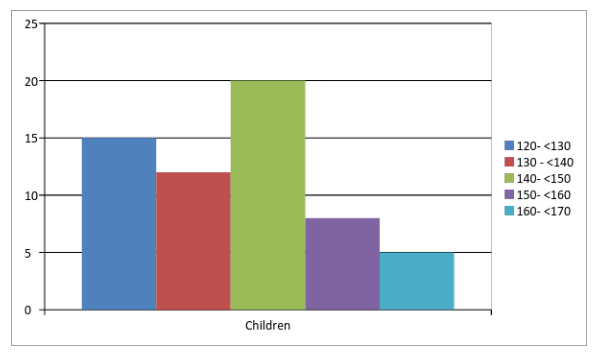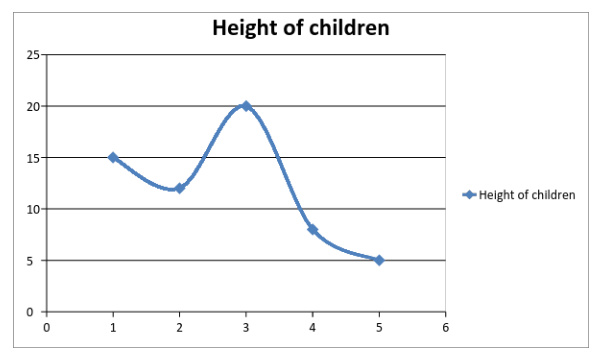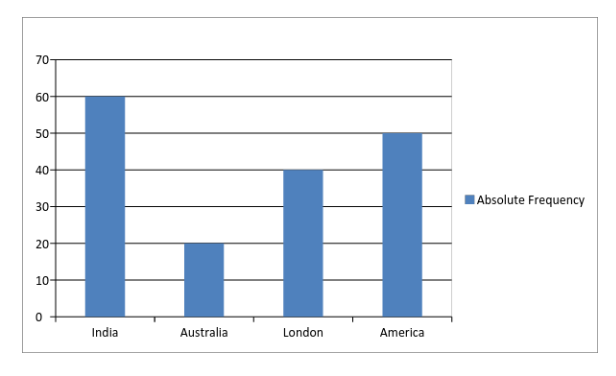# Graphical Methods for Describing Data

GRE tests the skills of aspirants through a variety of questions ranging from algebra to data analysis. Data analysis is considered to be an important topic in the GRE syllabus. There are many branches of Data Analysis, and one of those methods is through graphs which can be used for depicting the data. Let us understand the graphical method for describing data.

There are many ways through which the organization and summarization of data can be done. Even though the tabular form is the most famous method for representing the data, but there are other methods as well that are no less famous, these methods include; graphical method and numerical methods. The correct method of representation depends on the fact that whether the data is numerical or non- statistical in nature.

In this article, we will get to know the topics that come under the category of graphical methods for describing data in the syllabus of Data Analysis in GRE.

• Frequency Distribution: Frequency distribution is either a table or a graph that represents numerical values or various categories along with their associated frequencies.
• Pie Graphs: It is also known as circle charts, and is used for presenting a limited number of classes. It represents how a whole can be represented in sub-sections. Each part of a pie chart is known as a section, and the entire chart makes an angle of 360 degrees.
• Bar Chart: Bar graph is the most commonly used graphical display for representing counts or frequencies. Rectangular bars are used for sorting the data, and the height of each rectangular bar is in proportion to the incidence of the data. All the bars are of the same width and are either vertically or horizontally represented.
• Segmented Bar charts: This is a subcategory of the bar charts. This graph is used for representing various subcategories or subgroups. In this, each bar is divided into segments that represent different subgroups, in which the height of each section is in proportion to the relative frequency of the subset that is represented by the segments.
• Scatterplots: Line Graphs and scatter plots are almost similar to one another. Scatter plots mainly show how much one variable gets affected by other variables. The relationship between two variables is known as correlation.

Commonly, it consists of huge amount of data. Hence, nearer the data points are while plotting the graph to make a straight line, higher will be the correlation among the variables, that is, stronger will be the relationship.

• Time Series Plots: A time series plot is a graphical representation through which you can evaluate patterns and its behavior over a period. In a time series plot, observation is displayed against the y-axis and time intervals are present on the x-axis. It is also known as time plots.
• Histograms: Histograms are used for representing data that are divided in equal frequencies. There is no space between two columns and the bars show continuous data.

Let us take an example and understand each graphical method in detail.

Question: There is a survey conducted in which data of about 60 children are collected. The data is as follows: Number of children in the height range of

120 to less than 130 cms are 15

130 to less than 140 cms are 12

140 to less than 150 cms are 20

150 to less than 160 cms are 8

160 to less than 170 cms are 5

Solution:

Frequency Distribution

Height of children (in cms) Absolute frequency Relative Frequency
120 to less than 130 15 25%
130 to less than 140 12 20%
140 to less than 150 20 33.33%
150 to less than 160 8 13.33%
160 to less than 170 5 8.33%
Total 60 100 %

Pie ChartHistogramsScatterplotsBar Graph: Let us take an example to understand it:

 Birthplace of Children Absolute Frequency Relative Frequency India 60 35.29% Australia 20 11.76% London 40 23.52% America 50 29.41% Total 170 100%

So the Bar Graph will look like:BYJU’S will be glad to help you in your GRE preparation journey. You can ask for any assistance related to GRE from us by just giving a missed call at +918884544444, or you can drop an SMS. You can write to us at gre@byjus.com.